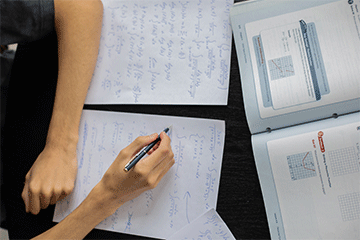NEWS

# Practical proofs

## David Varner, a graduate student in the Math department, completed research on how to improve the pedagogy of mathematical proofsDavid Varner, a graduate student in the Math Department, completed his research on the pedagogy of mathematical proofs. Proofs serve as the explanation for how mathematical formulas function and create the foundation for math. Varner’s research works to make sure that proofs are taught in a way that makes them understandable for math students.

Despite being the foundation of mathematics, most students don’t see proofs in the classroom until college. The lack of prior knowledge of proofs can create issues for students, as they are the basis of advanced mathematics. Under the supervision of Katrina Piatek-Jimenez, Varner took four proofs out of various math textbooks, and gave them to three math professors, asking them to improve them as if they were for beginning students. These revised proofs were then given to seven math students.

Varner then asked the professors why they made the changes they did and asked the students what helped and didn’t help. Most of the alterations followed the Communities of Practice framework, which approaches teaching through the lens that students learn by doing. A frequent issue when teaching advanced proofs is that they are dependent on having prior knowledge of certain ideas and formulas. Breaking down this knowledge makes the proofs more accessible to beginning students.

From the student perspective, it was found that the most appreciated change was an increase in exemplified work. Students responded that they could look up definitions and play around with formulas but providing a way for them to check their application was very helpful. It was also found that reformatting proofs to make sure the layout matched the steps they needed to follow made following along much easier.

Moving forward, Varner hopes to expand this research, using larger sample groups and looking into a more causal relationship. He also wants to expand on the Communities of Practice framework in mathematical higher education, as this practice isn’t frequently applied to math.